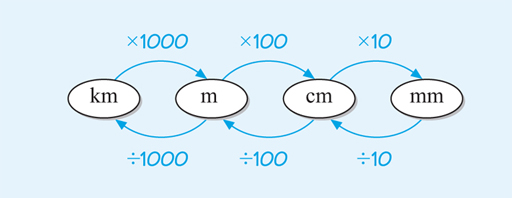Science, Maths & Technology

### Become an OU studentSucceed with maths: part 2

Start this free course now. Just create an account and sign in. Enrol and complete the course for a free statement of participation or digital badge if available.

# 3 Length: SI units

Length is one of the most common measurements that is used every day. This can tell you how far away the nearest town is, the width of a fridge or your height. In science it can be used on very different scales to measure the size of the universe, or at the other extreme, the diameter of an atom.

The base unit for length in the SI is the metre, abbreviated with a lower case m. An upper case M has a very different meaning, it is the prefix for a million times larger, so care needs to be taken with this! For those of you who are more familiar with imperial measurements, a metre is very roughly the same size as a yard.

Putting the metre together with the prefixes covered in the previous section gives:

• kilometre (km) – one thousand times bigger than a metre
• centimetre (cm) – a hundredth the size of a metre
• millimetre (mm) – a thousandth of the size of a metre.

This not only gives an idea of the size of these units but also how they relate to each other; that is, how many centimetres and millimetres there are in a metre and how many metres there are in a kilometre. This is important knowledge for when you want to change between units – usually known as converting.

So, if one centimetre is a hundredth of a metre, that means one metre must contain 100 centimetres. Similarly, one metre contains 1000 millimetres and one kilometre is the same as 1000 metres.

This can be summarised as follows:

• 1 km = 1000 m
• 1 m = 100 cm
• 1 m = 1000 mm.

or in a diagram that shows how to change between units, as in Figure 1 below:Figure 1 Relationships between SI units of length

This should make sense from what has been established so far about how the different units are connected.

For example, looking at kilometres, you know that there are 1000 m in 1 km, so in 2 km there will be 2000 m or 2 x 1000 m. So, to change from kilometres to metres you just need to multiply by 1000, as shown in Figure 1.

You’ll look at how to convert between different SI units of length in more detail in the next section.